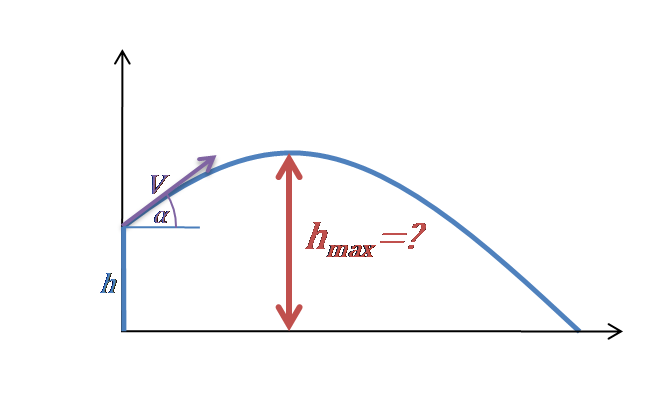# Maximum Height Calculator - Projectile Motion

Created by Hanna Pamuła, PhD candidate
Reviewed by Bogna Szyk
Last updated: May 20, 2020

The maximum height calculator is a tool for finding the maximum vertical position of a launched object in projectile motion. Whether you need the max height formula for an object starting directly off the ground or from some initial elevation - we've got you covered. If you're still wondering how to find maximum height of a projectile, read the two short paragraphs below, and everything should become clear.

## How to find the maximum height of a projectile?

Maximum height of the object is the highest vertical position along its trajectory. The object is flying upwards before reaching the highest point - and it's falling after that point. It means that at the highest point of projectile motion, the vertical velocity is equal to 0 (Vy = 0).

0 = Vy – g * t = V₀ * sin(α) – g * th

From that equation we can find the time th needed to reach the maximum height hmax:

th = V₀ * sin(α) / g

The formula describing vertical distance is:

y = Vy * t – g * t² / 2

So, given y = hmax and t = th, we can join those two equations together:

hmax = Vy * th – g * th² / 2

hmax = V₀² * sin(α)² / g – g * (V₀ * sin(α) / g)² / 2

hmax = V₀² * sin(α)² / (2 * g)

And what if we launch a projectile from some initial height h? No worries! Apparently, the calculations are a piece of cake - all you need to do is add this initial elevation!

hmax = h + V₀² * sin(α)² / (2 * g)

Let's discuss some special cases with changing angle of launch:

• if α = 90°, then the formula simplifies to:

hmax = h + V₀² / (2 * g)  and the time of flight is the longest.

If, additionally, Vy = 0, then it's the case of free fall. Also, you may want to have a look at our even more accurate equivalent - the free fall with air resistance calculator.

• if α = 45°, then the equation may be written as:

hmax = h + V₀² / (4 * g) and in that case, the range is maximal if launching from the ground (h = 0).

• if α = 0°, then vertical velocity is equal to 0 (Vy = 0), and that's the case of horizontal projectile motion. As sine of 0° is 0, then the second part of the equation disappears, and we obtain :

hmax = h - initial height from which we're launching the object is the maximum height in projectile motion.

## Maximum height calculator helps you find the answer

Just relax and look how easy-to-use this maximum height calculator is:

1. Choose the velocity of the projectile. Let's type 30 ft/s.
2. Enter the angle. Assume we're kicking a ball ⚽ at an angle of 70°.
3. Optionally, type the initial height. In our case, our starting position is the ground, so type in 0. Can the ball fly over a 13-ft fence?
4. Here it is - maximum height calculator displayed the answer! It's 12.35 ft. So it will not fly over the mentioned barrier - throw it harder or increase the angle to reach your goal.

Just remember that we don't take air resistance into account!

Hanna Pamuła, PhD candidate
Velocity
ft/s
Angle of launch
deg
Initial height
ft
Maximum height
Maximum height
ftPeople also viewed…

### BMR - Harris-Benedict equation

Harris-Benedict calculator uses one of the three most popular BMR formulas. Knowing your BMR (basal metabolic weight) may help you make important decisions about your diet and lifestyle.

### Capacitor code

Try the capacitor calculator if you want to find the meaning of the capacitor code and the value of its capacitance. You can also evaluate what is the charge stored in the capacitor for a specific voltage.

### Secretary problem (Valentine's day)

Use the dating theory calculator to enhance your chances of picking the best lifetime partner.

### Shear wave velocity

Use the shear wave velocity calculator to determine the velocity of a shear wave propagating in the body.